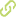# A deterministic alternative to competent genetic algorithms that solves to optimality linearly decomposable non-overlapping problems in polynomial time Chapter in Scopus•
• Overview
•
•
• View All
•

### Abstract

• David Goldberg has defined a competent genetic algorithm as one which "can solve hard problems, quickly, accurately, and reliably." Among other competent genetic algorithms that have been developed are the Bayesian optimization algorithm (BOA), the fast messy genetic algorithm (fmGA), and the linkage learning genetic algorithm (LLGA). These algorithms have been tested on problems of bounded difficulty that are additive separable formed by deceptive subproblems of order not greater than k, where k < ¿ BOA, fmGA, LLGA, and other competent genetic algorithms are stochastic, and thus, can only be assured of attaining optimality in a probabilistic sense. In this paper, we develop a deterministic algorithm that solves to optimality all linearly decomposable problems in a polynomial number of function evaluations with respect to the maximum size of the subproblems, k. The algorithm presented does not rely on a population, does not recombine individuals or apply any other genetic operator. Furthermore, because it is deterministic, the number of function evaluations required to find the optimum can be known in advance. The algorithm presented solves both the linkage and the optimization problems by finding the disjoint sets of related variables and the optimal values of these variables at the same time. The fact that such an algorithm can be devised has important implications for the design of GA-hard problems, and the development and evaluation of genetic optimization algorithms. © Springer-Verlag Berlin Heidelberg 2005.

### Publication date

• December 1, 2005